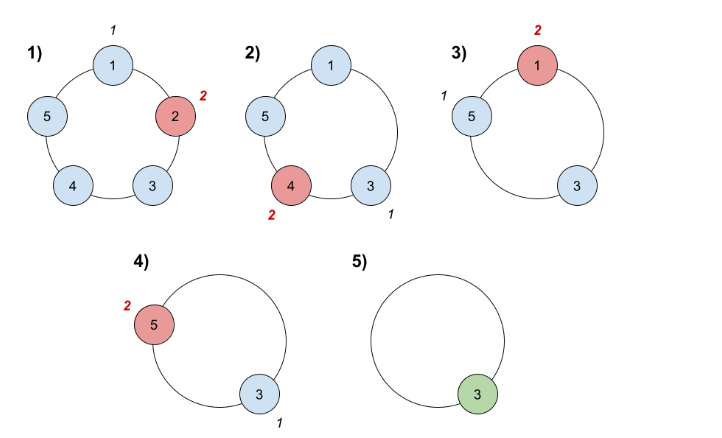## Given problem

There are n friends that are playing a game. The friends are sitting in a circle and are numbered from `1` to `n` in `clockwise` order. More formally, moving clockwise from the `ith` friend brings you to the `(i+1)th` friend for `1 <= i < n`, and moving clockwise from the `nth` friend brings you to the `1st` friend.

The rules of the game are as follows:

1. Start at the `1st` friend.
2. Count the next `k` friends in the clockwise direction including the friend you started at. The counting wraps around the circle and may count some friends more than once.
3. The last friend you counted leaves the circle and loses the game.
4. If there is still more than one friend in the circle, go back to step `2` starting from the friend `immediately clockwise` of the friend who just lost and repeat.
5. Else, the last friend in the circle wins the game.

Given the number of friends, `n`, and an integer `k`, return the winner of the game.

Example 1:``````Input: n = 5, k = 2
Output: 3
Explanation: Here are the steps of the game:
1) Start at friend 1.
2) Count 2 friends clockwise, which are friends 1 and 2.
3) Friend 2 leaves the circle. Next start is friend 3.
4) Count 2 friends clockwise, which are friends 3 and 4.
5) Friend 4 leaves the circle. Next start is friend 5.
6) Count 2 friends clockwise, which are friends 5 and 1.
7) Friend 1 leaves the circle. Next start is friend 3.
8) Count 2 friends clockwise, which are friends 3 and 5.
9) Friend 5 leaves the circle. Only friend 3 is left, so they are the winner.
``````

Example 2:

``````Input: n = 6, k = 5
Output: 1
Explanation: The friends leave in this order: 5, 4, 6, 2, 3. The winner is friend 1.
``````

Constraints:

• 1 <= k <= n <= 500

The first approach is to use brute force algorithm. It means that we will create a circular linked list to simulate all steps above.

Below is the source code of this way.

``````class Solution {
public int findTheWinner(int n, int k) {

for (int i = 1; i <= n; ++i) {
circularGame.insert(i);
}

int count = k;
while (circularGame.hasNotRemainedOne()) {
currentNode = kNode.next;

circularGame.remove(kNode);
}

}

private Node tail;

public void insert(int value) {
Node newNode = new Node(value);

} else {
this.tail.next = newNode;
}

this.tail = newNode;
}

public void remove(Node node) {
if (node == null) {
return;
}

do {
if (currentNode.next == node) {
currentNode.next = node.next;

}

if (this.tail == node) {
this.tail = currentNode;
}

break;
}

currentNode = currentNode.next;
}

public boolean hasNotRemainedOne() {
}

public Node incrementWithSteps(Node startNode, int k) {
if (k == 1) {
return startNode;
}

if (k < 1 || startNode == null) {
return null;
}

Node currentNode = startNode;
do {
currentNode = currentNode.next;
--k;
} while (k != 1);

return currentNode;
}

}

static class Node {
public Node next;
public int value;

public Node(int value) {
this.value = value;
this.next = null;
}
}

}
}
``````

The complexity of this solution:

• Time complexity: O(n)
• Space complexity: O(n)

## Using Queue

To reduce the number of line of code in the above solution, we can use Queue data structure. The logic is still remained.

``````class Solution {
public int findTheWinner(int n, int k) {

for (int i = 1; i <= n; ++i) {
}

while (circularGame.size() > 1) {
int count = k;

while (count > 1) {
int current = circularGame.poll();

--count;
}

circularGame.poll();
}

return circularGame.peek();
}
}
``````

## Wrapping up

• When running the two solutions in the leetcode, we found that:

• The way that use CircularLinkedList is running faster than Using Queue data structure, because using Queue took 125 ms, but Using Circular Linked List took 8 ms.

Reference:

1823. Find the Winner of the Circular Game